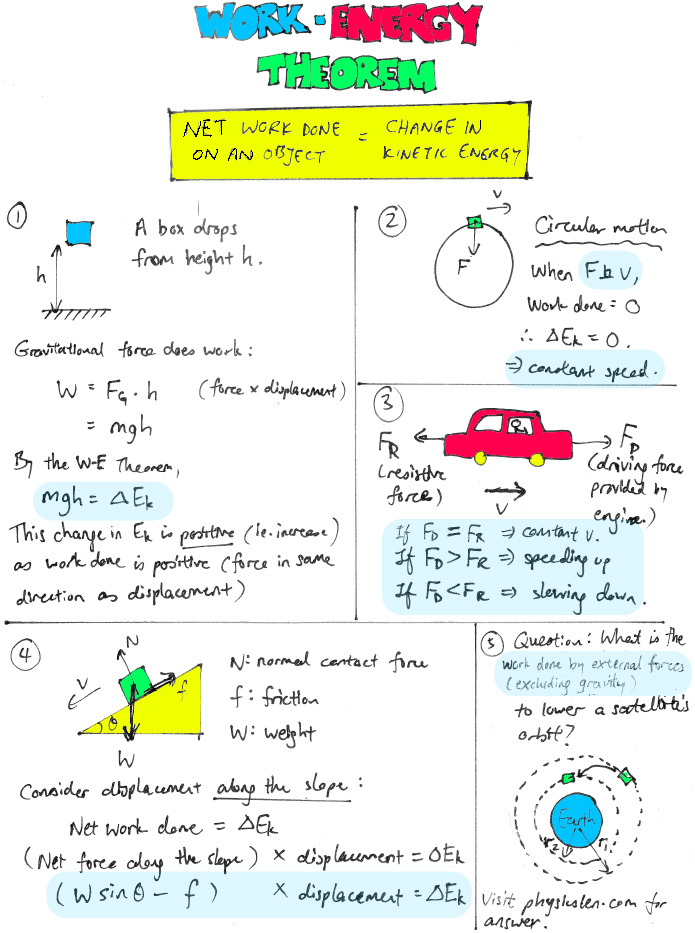# Work-Energy Theorem – key conceptsSince $E_k=\dfrac{GMm}{2r}$ for satellites in orbit (where G is the gravitational constant, r is the radius of the orbit, M is the mass of the planet and m is the mass of the satellite), kinetic energy increases. Hence $\Delta {E_k}=GMm(\dfrac{1}{2r_2}-\dfrac{1}{2r_1})$ is positive.
Work done by gravity is positive as the radial displacement is in the same direction as that of the force. Work done by gravity = $-\Delta{E_p}=-[-GMm(\dfrac{1}{r_2}-\dfrac{1}{r_1})]=2\times\Delta{E_k}$
According to the Work-Energy Theorem, net work = work done by gravity + work done by external force = $\Delta {E_k}$. Hence, work done by external force = $-\Delta{E_k}$.